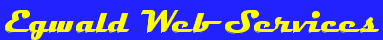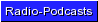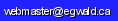Egwald Mathematics: Optimal Control by Elmer G. Wiens Egwald's popular web pages are provided without cost to users. Please show your support by joining Egwald Web Services as a Facebook Fan:Follow Elmer Wiens on Twitter:Applications of Optimal Control Theory Using the Pontryagin Maximum Principle. The following optimal control theory web pages solve some of the standard problems of optimal control. The solutions to the optimal control problems are presented diagrammatically. On these interactive web pages, you can see how the solutions depend on the initial conditions of the problems. 1. Learn how to use the Pontryagin Maximum Principle to solve the rocket car optimal control theory problem. This solution to this optimal control theory problem shows the optimal firing sequence for the rockets mounted at the front and rear of a rocket car that will bring the rocket car to rest at a designated position in the minimum amount of time. I explain and show in diagrams the interaction of the state and adjoint (co-state) variables in the optimal time path of the rocket car. The attainable set and the controllability matrix are also defined and explained. 2. Shoot down a scud missile with a shoulder mounted rocket: Intercept Missile problem. By applying Pontryagin's Maximum Principle to the nonlinear system of equations of motion of the scud missile and shoulder mounted rocket, I derive the optimal control theory minimum time solution to intercepting the scud missile. 3. Solve the famous linearized pendulum (harmonic oscillator) problem using optimal control theory. 4. Solve Linear-Quadratic Optimal Control problems with a finite or infinite time horizon: Linear-Quadratic Regulator. I solve finite time horizon optimal control problems using the Matrix Ricatti Equation, and infinite time optimal control problems using the Algebraic (Steady-State) Ricatti Equation.Copyright © Elmer G. Wiens:   Egwald Web ServicesAll Rights Reserved.    Inquiries